Partition Table Records

A record in a hard disk's partition table contains 16 bytes divided into 6 fields. The structure is as follows: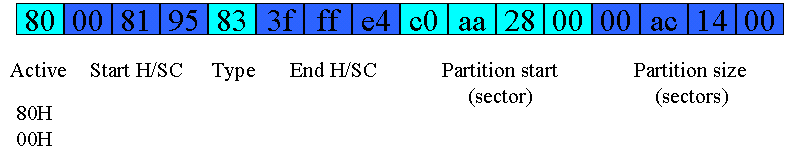The partition represented above is the same one represented as /dev/hda3 (the 3rd partition) in the following output from the fdisk utility: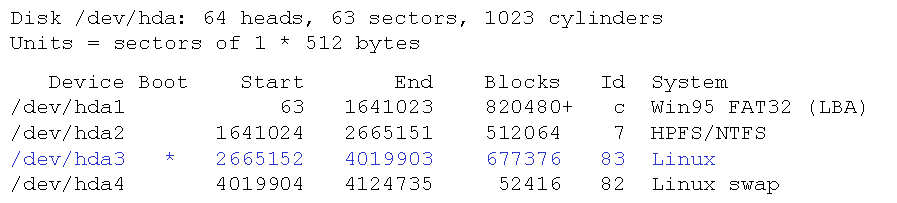Note the following correspondences: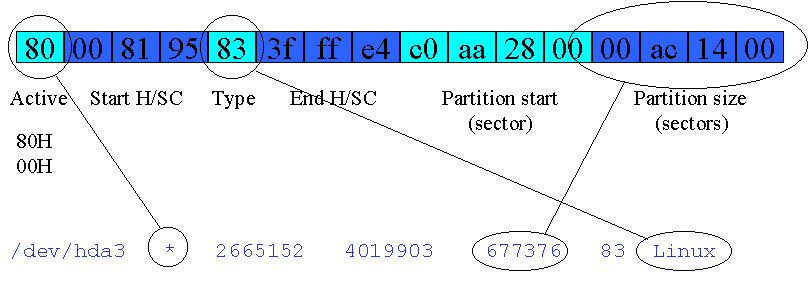First correspondence - the value of the "active" field can be either 80H or 00H (H indicates the numbers are presented using the base 16 or "hexadecimal" number system). If 00H, the fdisk display shows blank space, if 80H it shows an asterisk.

Second correspondence - the "type" field contains a code number. There is a code number for each possible physical filesystem type in which the partition might be formatted. In the example, it is indicated that 83H is the code assigned to represent the Linux (ext2) filesystem type. There is a large number of other codes and corresponding filetypes.

Third correspondence - the "partition size" field contains the number of sectors represented using 4 2-digit hexadecimal numbers, each of which is the value in one place of a 4-place base-256 number. An example should clarify. The 4-digit base-10 number 5826 appears below, indicating how the value of the number is derived from the values of its 4 digits.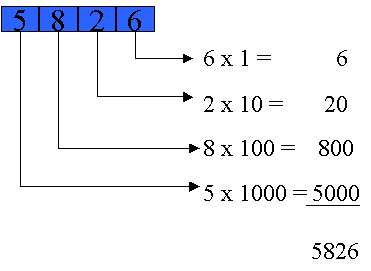Everybody knows that 5826 means five-thousand eight-hundred twenty-six. But only because they have been taught that 1, 10, 100, and 1000 are part of the calculation even though they are never written. All that is actually written is a total of twenty-one (5 and 8 and 2 and 6). Only the interpretation that people supply, which is purely mental and unwritten, informs what is written with its intended value.

The above partition size field represents its value similarly, with a few differences. First difference, the "digits" in this base-256 number are not themselves represented with base-256 digits (nobody has invented that many digits), but with hexadecimal numbers instead. Second difference, the number system is base 256 instead of base 10. Therefore, the place-values are powers of 256 instead of 10. Rather than 1, 10, 100, and 1000 they are instead 1, 256, 65536, and 16777216. Third difference, the order of significance is reversed. Instead of the weightier digits written to the left and descending in significance with rightward position, the least weighty digit appears at the left and digits increase in significance with rightward position. In the above decimal example, the 5 is the heavyweight, next comes the 8, then the 2, and the 6 is the lightweight. In the partition table size field its just the opposite: the 00 at the left is lightweight, the ac is heavier, the 14  heavier still, and the rightmost 00 is the heavyweight champion within the number.

If adjustments are made for these differences, the correspondence between the value contained in the partition size field (00 aa 14 00), and the partition's size as reported by fdisk (677376), can be reconciled.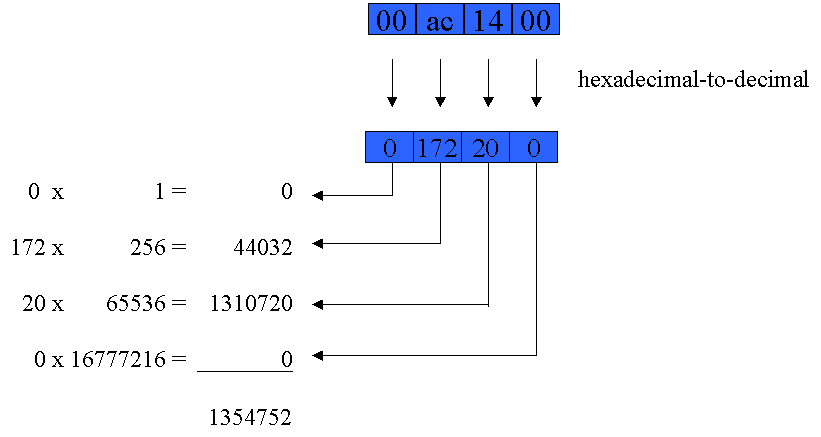The size of the partition is thus 1,354,752 sectors. fdisk reports that the size of the sector is 677376 blocks. 677376 is only half of 1,354,752. But because a sector is 512 bytes while a block is 1024, the two reported sizes are the same.

Detailed information about partition tables and records can be found at